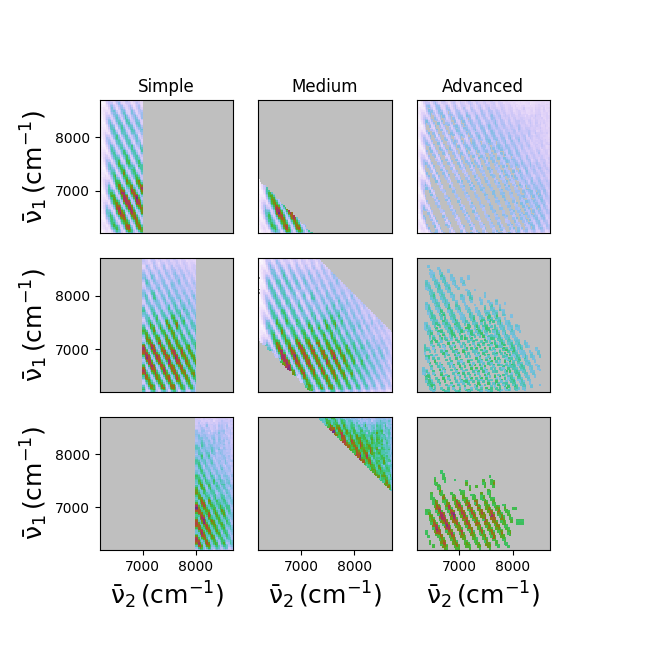Split¶

Some examples of how splitting works.Out:

Correction factor applied to d1
Correction factor applied to d2
data created at /tmp/a6nw6i_0.wt5::/
axes: ('w2', 'w1')
shape: (81, 81)
axis w2 converted from wn to wn
axis w1 converted from wn to wn
variable w1 converted from wn to wn
variable w2 converted from wn to wn
variable wm converted from nm to wn
split data into 3 pieces along <w2>:
0 : -inf to 7000.00 wn (26, 1)
1 : 7000.00 to 8000.00 wn (32, 1)
2 : 8000.00 to inf wn (23, 1)
axis w1+w2+7000 converted from wn to wn
split data into 3 pieces along <w1+w2+7000>:
0 : -inf to 20400.00 wn (33, 33)
1 : 20400.00 to 23000.00 wn (81, 81)
2 : 23000.00 to inf wn (45, 45)
split data into 3 pieces along <strange>:
0 : -inf to 0.20 None (81, 81)
1 : 0.20 to 0.40 None (71, 76)
2 : 0.40 to inf None (54, 46)


from matplotlib import pyplot as plt
import WrightTools as wt
from WrightTools import datasets

d = wt.data.from_PyCMDS(datasets.PyCMDS.w2_w1_000)

d.convert("wn", convert_variables=True)

# A simple split along an axis
a = d.split("w2", [7000, 8000])
# A more complicated split along some diagonal
b = d.split("w1+w2+7000", [20400, 23000], units="wn")
# A particularly strange split
d.create_variable("strange", values=d.channels.points / d.channels.max())
c = d.split("strange", [.2, .4])

# Plot the splits in columns
fig, gs = wt.artists.create_figure(nrows=len(c), cols=[1, 1, 1])
for j, (col, title) in enumerate(zip([a, b, c], ["Simple", "Medium", "Advanced"])):
for i, da in enumerate(col.values()):
ax = plt.subplot(gs[i, j])
if i == 0:
ax.set_title(title)
ax.pcolor(da)
ax.set_xlim(d.axes.min(), d.axes.max())
ax.set_ylim(d.axes.min(), d.axes.max())

wt.artists.set_fig_labels(xlabel=d.axes.label, ylabel=d.axes.label)


Total running time of the script: ( 0 minutes 5.087 seconds)

Gallery generated by Sphinx-Gallery